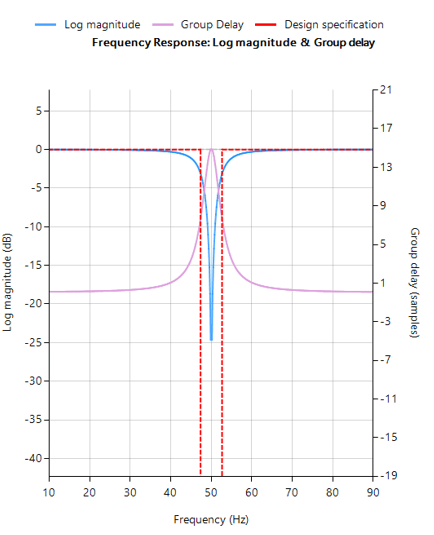# Notch Filter

A Notch Filter is also known as a Band Stop filter or Band Reject Filter. The filter passes the signals above and below a specified frequency band unaltered. This band is called the stop band frequency range. And it attenuates (‘rejects’) the frequencies within this range to very low levels.Syntax
Hd = notch (Fo, BW, DFormat)

Description
IIR notch filter design, defined as:

$$H(z)=\frac{1-2cos⁡ w_o z^{-1}+z^{-2}}{1-2rcos⁡ w_o z^{-1}+ r^2 z^{-2}}$$

where, $$w_o=\frac{2\pi f_o}{f_s}$$ controls the centre frequency, $$f_o$$ of the notch, and $$r=1-\frac{\pi BW}{f_s}$$controls the bandwidth (-3dB point) of the notch.

Fo: centre frequency of the notch

BW: Bandwidth (-3dB point) of the notch. Where, $$BW ≤ \frac{F_o}{4}$$

DFormat: allows you to specify the display format of resulting digital filter object.

 symbolic Display a symbolic representation of the filter object. If the order > 10, the symbolic display option will be overridden and set to numeric numeric Display a matrix representation of the filter object void Create a filter object, but do not display output

Example

ClearH1;  // clear primary filter from cascade
ShowH2DM;   // show DM on chart

interface BW={5,40,2,10};

Main()

F=75;
Hd=notch(F,BW,"symbolic");

Num = getnum(Hd); // define numerator coefficients
Den = getden(Hd); // define denominator coefficients
Gain = getgain(Hd); // define gain